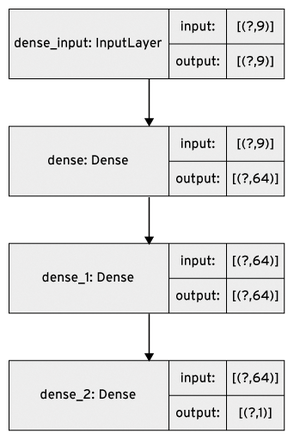# On the Edge

#### Lettuce Weight Regression Model

A regression problem aims to model the output of a continuous value, such as temperature or weight. In contrast, a classification problem aims to select a class from a list of classes.

To build the model, I used the new tf.keras API introduced in TensorFlow 2.0. The goal is to predict the lettuce fresh weight at a certain date after planting. To set up the R&D environment on the computer, I used Python 3.7, created a virtual environment with `virtualenv` , and installed the TensorFlow distribution with `pip` (Listing 1).

Listing 1

TensorFlow Installation

```python3 --version
pip3 --version
virtualenv --version
virtualenv --system-site-packages -p python3 ./venv
## Activate the environment
source ./venv/bin/activate
## Install the TensorFlow distribution
pip install --upgrade tensorflow=2.0 pandas numpy pathlib
## Check the setup
python -c "import tensorflow as tf;print(tf.reduce_sum(tf.random.normal([1000, 1000])))"```

#### Neural Network

For simplicity and easy comprehension, consider the 10 features shown in Table 1 with sample data values. Note that I am working on real-world data from sensors that could fail and need to be calibrated. To avoid aberrant results, then, it is crucial to implement strong preprocessing techniques like sampling, filtering, and normalization before feeding the data into the neural network.

Table 1

Sample Dataset

Feature Description Sample Value
Weight Weight of lettuce head 135.0 g
DaP Days after planting 10 days
CumCO2 Cumulative sum of CO2 4 ppm
CumLight Cumulative sum of light 1420.0 umol/sq m/sec
CumTemp Cumulative sum of temperature 8623.0°C
LeafArea Leaf area 156 sq cm
Irrigation system (one-hot encoding)
NFT Hydroponic nutrient film 0.0
HPA High-pressure aeroponics 1.0
Ebb&Flood Hydroponic ebb and flood 0.0
Nebu Nebulized aeroponics 0.0

The dataset is split in two for training and testing. The value to be predicted is the weight, so the remaining nine features are separated out to be the input of the neural network (i.e., weight will be the output).

To build the model, I use a simple Sequential model (Listing 2) with two densely connected hidden layers and an output layer that returns a single continuous value: the lettuce fresh weight. I chose only two hidden layers, because there is not much training data and I only have nine features. In such cases, a small network with few hidden layers is better to avoid overfitting. I empirically chose the widely used rectified linear unit (ReLU) activation function and the mean squared error (MSE) loss function for regression problems (different loss functions are used for classification problems).

Listing 2

Sequential Model

```01 import tensorflow as tf
02 [...]
03
04 model = tf.keras.Sequential([
05     tf.keras.layers.Dense(64, activation=tf.nn.relu, input_shape=[len(train_dataset.keys())]),
06     tf.keras.layers.Dense(64, activation=tf.nn.relu),
07     tf.keras.layers.Dense(1)
08 ])
09
10 optimizer = tf.keras.optimizers.RMSprop(0.001)
11 model.compile(loss='mean_squared_error',
12    optimizer=optimizer,
13    metrics=['mean_absolute_error', 'mean_squared_error'])```

The model is shown in Figure 4. Note that the number of inputs to the neural network is nine, which corresponds to the number of input features. Now, the model can be trained by the training dataset:Figure 4: The last nine features in Table 1 serve as input for the sequential model. The first feature (weight) is the output.
`model.fit(train_features, train_weights, epochs=100, validation_split=0.2, verbose=0)`

After training, when I save and export the trained model (Listing 3), I see that it is 86077 bytes.

Listing 3

Exporting the Model

```01 model_export_dir= "./models/lg_weight/"
02 tf.saved_model.save(model, model_export_dir)
03 root_directory = pathlib.Path(model_export_dir)
04 tf_model_size = sum(f.stat().st_size for f in root_directory.glob('**/*.pb') if f.is_file())
05 print("TF model is {} bytes".format(keras_model_size))```

#### Convert and Optimize

Now comes the important part of this tutorial: converting and saving the trained model to the TensorFlow Lite format with the default optimizations:

```converter = tf.lite.TFLiteConverter.from_keras_model(model)
tflite_model = converter.convert()
open('./models/model.tflite', "wb").write(tflite_model)```

Checking the model size, I see that it is now 20912 bytes, which is four times smaller than the original TensorFlow model:

```model_size = os.path.getsize('./models/model.tflite')
print("TFLite model is {} bytes".format(model_size))```

I can further reduce the model size with the optimizers offered by TensorFlow Lite Converter. The simplest form of post-training quantization quantizes only the weights from floating-point to 8 bits of precision, which is also called "hybrid" quantization:

```converter.optimizations = [tf.lite.Optimize.OPTIMIZE_FOR_SIZE]
tflite_quant_model = converter.convert()```

The model is now only 8672 bytes, which is 10 times smaller than the conventional TensorFlow model.

Express-Checkout as PDF
Price \$2.95
(incl. VAT)

SINGLE ISSUES

SUBSCRIPTIONS